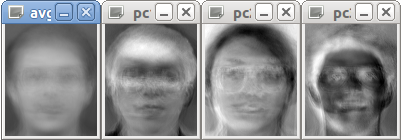# PCA in OpenCVThere are hardly any useful examples on how to do a Principal Component Analysis (PCA) with the OpenCV2 C++ API, at time of writing this. Maybe because it's simple for everyone else, but it took me some time to figure it out. So here is an example! The listing shows how to display the average image and the first three principal components.

``````/*
* Copyright (c) 2012. Philipp Wagner <bytefish[at]gmx[dot]de>.
* Released to public domain under terms of the BSD Simplified license.
*
* Redistribution and use in source and binary forms, with or without
* modification, are permitted provided that the following conditions are met:
*   * Redistributions of source code must retain the above copyright
*     notice, this list of conditions and the following disclaimer.
*   * Redistributions in binary form must reproduce the above copyright
*     notice, this list of conditions and the following disclaimer in the
*     documentation and/or other materials provided with the distribution.
*   * Neither the name of the organization nor the names of its contributors
*     may be used to endorse or promote products derived from this software
*     without specific prior written permission.
*
*/

#include <opencv2/core/core.hpp>
#include <opencv2/highgui/highgui.hpp>

#include <fstream>
#include <sstream>

using namespace cv;
using namespace std;

// Reads the images and labels from a given CSV file, a valid file would
// look like this:
//
//      /path/to/person0/image0.jpg;0
//      /path/to/person0/image1.jpg;0
//      /path/to/person1/image0.jpg;1
//      /path/to/person1/image1.jpg;1
//      ...
//
void read_csv(const string& filename, vector<Mat>& images, vector<int>& labels) {
std::ifstream file(filename.c_str(), ifstream::in);
if(!file)
throw std::exception();
std::string line, path, classlabel;
// For each line in the given file:
while (std::getline(file, line)) {
// Get the current line:
std::stringstream liness(line);
// Split it at the semicolon:
std::getline(liness, path, ';');
std::getline(liness, classlabel);
// And push back the data into the result vectors:
labels.push_back(atoi(classlabel.c_str()));
}
}

// Normalizes a given image into a value range between 0 and 255.
Mat norm_0_255(const Mat& src) {
// Create and return normalized image:
Mat dst;
switch(src.channels()) {
case 1:
cv::normalize(src, dst, 0, 255, NORM_MINMAX, CV_8UC1);
break;
case 3:
cv::normalize(src, dst, 0, 255, NORM_MINMAX, CV_8UC3);
break;
default:
src.copyTo(dst);
break;
}
return dst;
}

// Converts the images given in src into a row matrix.
Mat asRowMatrix(const vector<Mat>& src, int rtype, double alpha = 1, double beta = 0) {
// Number of samples:
size_t n = src.size();
// Return empty matrix if no matrices given:
if(n == 0)
return Mat();
// dimensionality of (reshaped) samples
size_t d = src.total();
// Create resulting data matrix:
Mat data(n, d, rtype);
// Now copy data:
for(int i = 0; i < n; i++) {
//
if(src[i].empty()) {
string error_message = format("Image number %d was empty, please check your input data.", i);
}
// Make sure data can be reshaped, throw a meaningful exception if not!
if(src[i].total() != d) {
string error_message = format("Wrong number of elements in matrix #%d! Expected %d was %d.", i, d, src[i].total());
}
// Get a hold of the current row:
Mat xi = data.row(i);
// Make reshape happy by cloning for non-continuous matrices:
if(src[i].isContinuous()) {
src[i].reshape(1, 1).convertTo(xi, rtype, alpha, beta);
} else {
src[i].clone().reshape(1, 1).convertTo(xi, rtype, alpha, beta);
}
}
return data;
}

int main(int argc, const char *argv[]) {
// Holds some images:
vector<Mat> db;

// Load the greyscale images. The images in the example are
// taken from the AT&T Facedatabase, which is publicly available
// at:
//
//      http://www.cl.cam.ac.uk/research/dtg/attarchive/facedatabase.html
//
// This is the path to where I stored the images, yours is different!
//
string prefix = "/home/philipp/facerec/data/at/";

// The following would read the images from a given CSV file
// instead, which would look like:
//
//      /path/to/person0/image0.jpg;0
//      /path/to/person0/image1.jpg;0
//      /path/to/person1/image0.jpg;1
//      /path/to/person1/image1.jpg;1
//      ...
//
// Uncomment this to load from a CSV file:
//

/*
vector<int> labels;
*/

// Build a matrix with the observations in row:
Mat data = asRowMatrix(db, CV_32FC1);

// Number of components to keep for the PCA:
int num_components = 10;

// Perform a PCA:
PCA pca(data, Mat(), CV_PCA_DATA_AS_ROW, num_components);

// And copy the PCA results:
Mat mean = pca.mean.clone();
Mat eigenvalues = pca.eigenvalues.clone();
Mat eigenvectors = pca.eigenvectors.clone();

// The mean face:
imshow("avg", norm_0_255(mean.reshape(1, db.rows)));

// The first three eigenfaces:
imshow("pc1", norm_0_255(pca.eigenvectors.row(0)).reshape(1, db.rows));
imshow("pc2", norm_0_255(pca.eigenvectors.row(1)).reshape(1, db.rows));
imshow("pc3", norm_0_255(pca.eigenvectors.row(2)).reshape(1, db.rows));

// Show the images:
waitKey(0);

// Success!
return 0;
}
``````